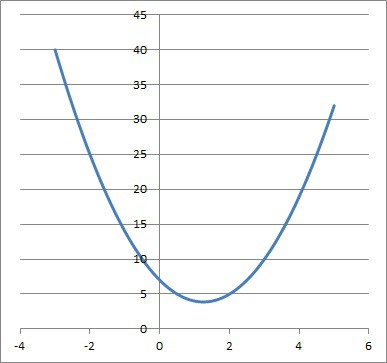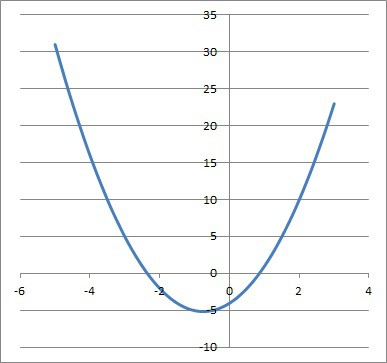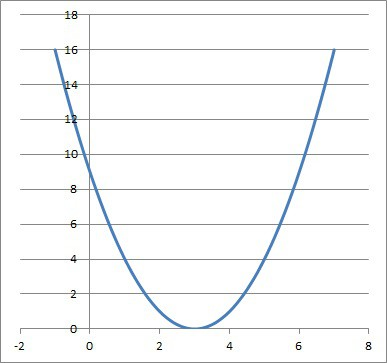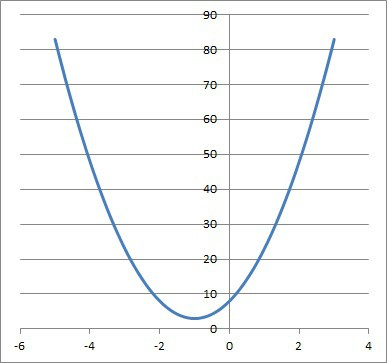# What Does Discriminant Mean In Math? (3 Key Ideas)

The discriminant is used throughout mathematics, from solving quadratic equations in elementary algebra to second order differential equations.  However, it is still necessary to know what the discriminant means and what it can tell us.

So, what does discriminant mean in math?  The discriminant is the expression b2 – 4ac, found under the radical in the quadratic formula. The discriminant classifies solutions of a quadratic equation: 2 real, 1 real repeated, or 2 complex conjugate. It also tells us if the corresponding parabola intersects the x-axis, and how it does so.

Of course, we can also look at the graph of a parabola and figure out the sign of the discriminant, based on where it intersects the x-axis.

In this article, we’ll talk about what the discriminant means in math, in the context of solving quadratic equations.  We’ll also look at some examples to make the concept clear.

Let’s begin.

## What Does Discriminant Mean In Math?

In math, the discriminant means the expression b2 – 4ac.  Sometimes, we use the letter D to stand for the discriminant, so D = b2 – 4ac.

Remember that the discriminant is the expression under the radical found in the numerator of the quadratic formula.

### What Does The Discriminant Tell You?

The discriminant is important in algebra because it tells us the nature of the solutions to a quadratic equation.  This distinction is made by the sign of the discriminant:

Remember that for each quadratic equation, we can draw the graph of a corresponding parabola (all of the points in the xy-plane that satisfy the equation).

The discriminant gives us some information about the parabola and what it looks like.  Specifically, the discriminant tells us whether the parabola intersects the x-axis, and how many times it does so.

Keep in mind that the points where the parabola intersects the x-axis are the values of x that satisfy the quadratic equation when it is set equal to zero.  These values are called solutions or roots of the equation.

### How Do You Find The Discriminant?

To find the discriminant of a quadratic equation, take the expression under the radical in the quadratic formula.

To evaluate the discriminant for a set of quadratic coefficients a, b, and c, all you need to do is substitute those values into the discriminant.

#### Example: How To Find The Discriminant

Let’s examine the quadratic equation 2x2 – 5x + 7 = 0.

Since it is of the form ax2 + bx + c = 0, we have a = 2, b = -5, and c = 7.

From the quadratic formula, we know that the expression for the discriminant is D = b2 – 4ac, so:

• D = b2 – 4ac
• D = (-5)2 – 4(2)(7)
• D = 25 – 56
• D = -31

In this case, our discriminant is D = -31.  Since D < 0 (negative discriminant), we know that:

• We will get two complex conjugate solutions to the quadratic equation (no real solutions).
• The graph of the corresponding parabola will not intersect the x-axis (the parabola lies entirely above the x-axis, since a > 0).This parabola is the graph of the quadratic y = 2x2 – 5x + 7. Note that it does not intersect the x-axis, since the discriminant is negative.

### How Do You Know If The Discriminant Is Positive?

Here are some ways to know that the discriminant is positive, based on the signs of a, b, and c:

• If a and c have opposite signs, then ac is negative, which means 4ac is negative, and -4ac is positive.  Since b2 is always positive or zero, then the discriminant b2 – 4ac will always be positive in this case.
• If c is zero and b is nonzero, then b2 is positive and 4ac is zero, so b2 – 4ac is positive.
• If a and c have the same sign, we must have b2 > 4ac in order for the discriminant to be positive.

#### Example 1: Positive Discriminant (Opposite Signs For a & c)

Consider the quadratic equation 2x2 + 3x – 4 = 0.

Since it has the form ax2 + bx + c = 0, we have a = 2, b = 3, and c = -4.

Note that a and c have opposite signs, since a is positive (a = 2) and c is negative (c = -4).

This means that -4ac will be positive (here, -4ac = -4(2)(-4) = 32).

So, b2 – 4ac will be positive (here, b2 – 4ac = 32 + 32 = 41).

So, the discriminant D = 41 is positive.This parabola is the graph of the quadratic y = 2x2 + 3x – 4. Note that it intersects the x-axis twice, since the discriminant is positive.

#### Example 2: Positive Discriminant (When c Is Zero)

Consider the quadratic equation 3x2 – 5x = 0.

Since it has the form ax2 + bx + c = 0, we have a = 3, b = -5, and c = 0.

Note that c is zero.

This means that -4ac will also be zero (4ac = 4(3)(0) = 0).

So, b2 – 4ac = b2 will be positive, regardless of the sign of b (here, b2– 4ac = b2 = (-5)2 = 25).

So, the discriminant D = 25 is positive.

#### Example 3: Positive Discriminant (Same Sign For a & c, with b2 > 4ac)

Consider the quadratic equation 4x2 – 15x + 12 = 0.

Since it has the form ax2 + bx + c = 0, we have a = 4, b = -15, and c = 12.

Note that a and c have the same sign, since a is positive (a = 4) and c is positive (c = 12).

This means that -4ac will be negative (here, -4ac = -4(4)(12) = -192).

However, b2 is large enough that b2 – 4ac will still be positive (here, b2 – 4ac = (-15)2 – 192 = 225 – 192 = 33).

So, the discriminant D = 33 is positive.

### How Do You Know If The Discriminant Is Zero?

The discriminant can only be zero when b2 = 4ac.  There are a number of ways this can happen, but one way is when both b and c are zero (a can never be zero).

#### Example 1: Zero Discriminant (When b & c Are Zero)

Consider the quadratic equation 3x2 = 0.

Since it has the form ax2 + bx + c = 0, we have a = 3, b = 0, and c = 0.

Note that b and c are both zero.

This means that both b2 and 4ac will be negative, meaning b2 – 4ac = 0.

So, the discriminant D is zero.

#### Example 2: Zero Discriminant (When b & c Are Nonzero)

Consider the quadratic equation x2 – 6x + 9 = 0.

Since it has the form ax2 + bx + c = 0, we have a = 1, b = -6, and c = 9.

Note that b and c are both nonzero (b = -6, c = 9).

Here, b2 – 4ac = (-6)2 – 4(1)(9) = 36 – 36 = 0.

So, the discriminant D is zero.This parabola is the graph of the quadratic y = x2 – 6x + 9. Note that it intersects the x-axis once, since the discriminant is zero.

### How Do You Know If The Discriminant Is Negative?

Here are some ways to know that the discriminant is negative, based on the signs of a, b, and c:

• If a and c have the same sign, then ac is positive, which means 4ac is positive, and -4ac is negative.  If b is zero, then b2 is zero, and in that case, the discriminant b2 – 4ac will be negative.
• If a and c have the same sign, but b is not zero, then we must have b2 < 4ac in order to have a negative discriminant.

If c is zero, then the discriminant cannot be negative, since b2– 4ac reduces to b2, which is always positive or zero (since b is a real number).

This makes sense, since a value of c = 0 would give us:

• ax2 + bx + c = 0
• ax2 + bx + 0 = 0
• x(ax + b) = 0

This implies x = 0 or ax + b = 0 as solutions, which leaves us with two real solutions: x = 0 and x = -b/a.

#### Example 1: Negative Discriminant (Same Sign For a & c, With b Equal To Zero)

Consider the quadratic equation 3x2 + 7 = 0.

Since it has the form ax2 + bx + c = 0, we have a = 3, b = 0, and c = 7.

Note that a and c have the same sign, since a is positive (a = 3) and c is positive (c = 7).

We also have b = 0.

This means that -4ac will be negative (here, -4ac = -4(3)(7) = -84).

Also, b2 = 0.

So, b2 – 4ac will be negative (here, b2 – 4ac = 02 – 84 = -84).

So, the discriminant D = -84 is negative.

#### Example 2: Negative Discriminant (Same Sign For a & c, With b Not Zero)

Consider the quadratic equation 5x2 + 10x + 8 = 0.

Since it has the form ax2 + bx + c = 0, we have a = 5, b = 10, and c = 8.

Note that a and c have the same sign, since a is positive (a = 5) and c is positive (c = 8).

We also have b not equal to zero (b = 10).

This means that -4ac will be negative (here, -4ac = -4(5)(8) = -160).

So, b2 – 4ac will be negative (here, b2 – 4ac = 102 – 160 = 100 – 160 = -60).

So, the discriminant D = -60 is negative.This parabola is the graph of the quadratic y = 5x2 + 10x + 8. Note that it does not intersect the x-axis, since the discriminant is negative.

## Conclusion

Now you know what the discriminant means in the context of algebra in mathematics.  You also know when it can be positive, zero, or negative and what each of these cases tells you.